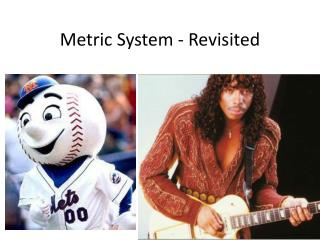DownloadDownload PresentationMetric System - Revisited

# Metric System - Revisited

Download Presentation## Metric System - Revisited

- - - - - - - - - - - - - - - - - - - - - - - - - - - E N D - - - - - - - - - - - - - - - - - - - - - - - - - - -
##### Presentation Transcript

1. Metric System - Revisited

2. Measuring – 3 things we measure Length Mass Volume

3. Two Methods English Metric

4. What you are used to. Inches, feet, & miles Length Pounds & Ounces English Mass Volume Quarts, pints, & gallons

5. What scientists use…and the rest of the world except Liberia and Burma. meters Length Mass grams Metric Volume liters

6. Now forget the left side of the bubble map… This is what you are to use from now on Metric System Length Mass Volume Meters (m) Grams (g) Liters (L)

7. These are the Suffixes…. Metric System Length Mass Volume Meters (m) Grams (g) Liters (L)

8. Suffixes (root word) cont… • The word prehistoric – “historic” is the suffix • It always comes after the prefix. • Same with multivitamin – “vitamin” is the suffix

9. Prefixes • They come before the suffix. • Prefixes by themselves don’t mean anything. • Example, the prefix “Un” means nothing • Added to unhappy means not happy.

10. Metric Prefixes • Kilo (k) – largest • Hecto (h) • Deca (da) • Deci (d) • Centi (c) • Milli (m) – smallest • They will never be by themselves, they have to be written with a suffix.

11. Prefixes + Suffixes for Mass (grams) • Prefix + Suffix = Term • Kilo (k) + gram (g)= Kilogram (kg) • Hecto (h) + gram(g) = Hectogram (hg) • Deca (da) + gram(g) = Decagram (dag) • Deci (d) + gram(g) = Decigram (dg) • Centi (c) + gram(g)= Centigram (cg) • Milli (m) + gram(g)= milligram (mg) • These terms only measure mass.

12. Prefixes + Suffixes for Volume(Liters) • Prefix + Suffix = Term • Kilo (k) + Liter (L) = Kiloliter (kL) • Hecto (h) + Liter(L) = Hectoliter (hL) • Deca (da) + Liter (L) = Decaliter (daL) • Deci (d) + Liter (L) = Deciliter (dL) • Centi (c) + Liter (L) = Centiliter (cL) • Milli (m) + Liter (L) = milliliter (mL) • These terms only measure volume.

13. Prefixes + Suffixes for length(meters) • Prefix + Suffix = Term • Kilo (k) + meter(m) = Kilometer (km) • Hecto (h) + meter(m) = Hectometer (hm) • Deca (da) + meter (m) = Decameter (dam) • Deci (d) + meter (m) = Decimeter (dm) • Centi (c) + meter (m) = Centimeter (cm) • Milli (m) + meter (m) = millimeter (mm) • These terms only measure meters.

14. Why do we have specific prefixes for each type of measurement… Length A big measurement Km hm/ dam dm/ cm A small one mm

15. Same scenario works for volume and mass • Volume – what units (prefix + suffix) would you use to measure the volume of this?_______ • Mass - what units (prefix + suffix) would you use to measure the mass of this?_________

16. How to measure volume • You measure the meniscus • Meniscus is the bottom of the dip of water.

17. Determining what each line may represent on a measuring tool. • Step 1 – find any two numbers… example 50 and 40 • Step 2 – subtract the two… 50 – 40 = 10 • Step 3 – count the lines in between them… start at the line above the bottom number, and stop at the line that is the top number. Example… from 40 to 50 I count 10 lines. • Step 4 – divide the answer from step 2 by the answer from step 3…. 10 divided by 10 = 1 • Your answer from step four tells you that each line equals 1. • This example the meniscus would read 43mL

18. How to measure length 10mm = 1cm 10cm = 1dm 10dm = 1m 10m = 1dam 10dam = 1hm 10hm = 1km 1cm 1mm

19. How to measure mass • Always measure in grams • As shown the balance is 373.3g • 300 + 70 + 3.3 = 373.3g

20. Celsius- standard form of temperature measurement Abbreviation C (Capitol) Human body is 98.6 F, and that is 37 C Metrics - Temperature

21. Great… How do I do conversions?

22. To remember • Kids • Have • Damaged • 3 • Different • Colorful • Millipedes

23. Great… How do I do conversions?Example #1: 0.250kg mg The decimal is moving

24. How do I do conversions?....contExample #1: 12mL LNote: If you have a whole number, the decimal is always at the end of the very right number. Example 12.0 The Decimal is moving